# Predicting The Direction Of A Reaction

## Introduction

When we deal with reactions that are reversible in nature, it is important to figure out the direction of the reaction at a given instance. For example, in the formation of ammonia at a commercial scale from nitrogen and hydrogen, we need to optimize the process for efficient production. Thus, it is important to predict the state of the reaction at any given instant.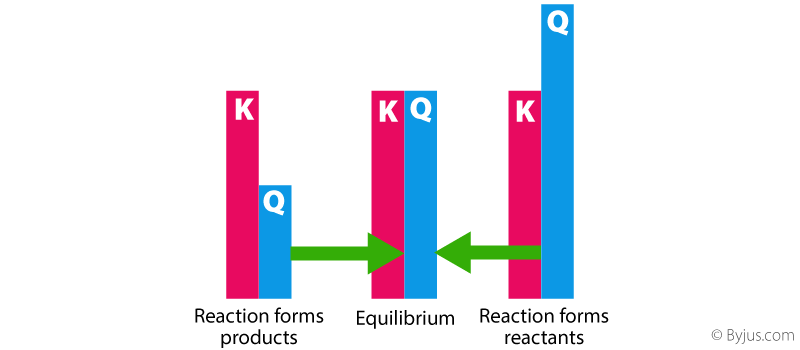As we know, the direction in which a given chemical reaction will proceed can be calculated using the knowledge of the reaction constant. In this section, we will learn about the prediction of the direction of the reaction at any stage using the value of the reaction quotient and the equilibrium constant.

## Calculating Reaction Quotient & Equilibrium Constant

Let us consider a chemical reaction given as under,

a A + b B ↔ c C + d D

The reaction quotient of the reaction can be calculated in terms of the partial pressure (Qp) and the molar concentration (Qc) in the same way as we calculate the equilibrium constant in terms of partial pressure (Kp) and the molar concentration (Kc) as given below.

### Calculating the Equilibrium Constant

The relationship between the products and the reactants in an equilibrium reaction is expressed with the help of the equilibrium constant (denoted by K). When the reaction is at equilibrium, the Kc for the reaction in terms of the molar concentration of the components can be given as,And Kp of the reaction in terms of the partial pressure of the components can be given as,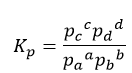### Calculating Reaction Quotient

The reaction quotient, often denoted by Q, provides a measure of the amount of reactant and product present at a given point during a chemical reaction. When the reaction is not at equilibrium, we write the reaction quotient of the reaction in terms of their molar concentration as,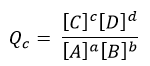And the reaction quotient in terms of the partial pressure of the components as,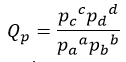## Predicting the Direction of a Chemical Reaction

From the above four expressions, the following three cases arise, depending upon the direction in which the reaction is expected to proceed.

• Q> Kc
In this case, the reaction proceeds in the reverse or the backward direction or in the direction of the reactants.
• Q< Kc
In this case, the reaction proceeds in the forward direction or the direction of the products.
• Q= Kc
In this case, the reaction is said to be at equilibrium and no net reaction occurs in any direction.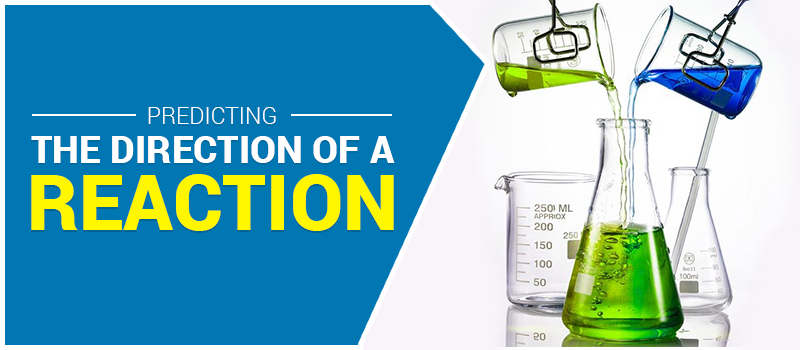The above result has been clearly shown in the figure given here. For any chemical reaction,

• If Qc>Kc,  the reaction goes from right to left.
• If Qc<Kc,  the reaction goes from left to right.
• If Qc=Kc,  the reaction is at equilibrium.